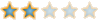Swapping digits in Four digit variableSwapping digits in Four digit variable

Code:
`class Gattu{static int swap(int a){        int e=a/1000;          //a=8719//        int g=a%1000;        //g=719//        int o=g/100;            //o=7//        int j=g%100;          //j=19//        int z=j*100;            //z=1900//        int y=e*10;            //o=70//        int w=o;                //w=8//        int v=z+y+w;        return(v);}        public static void main(String[] args){        int x=Integer.parseInt(args);        int p=Gattu.swap(x);        System.out.println("the answer is "+p);}}      `

Code:
`E:\Program Files\Java\jdk1.6.0\bin>java Gattu 8719the answer is 1987`shirish.vogga
New LSF MemberPosts : 3Re: Swapping digits in Four digit variable

wah!!!!!!!!!!!!!!
Shrish!!!!kiran
LSF RegularPosts : 46Permissions in this forum:
You cannot reply to topics in this forum